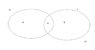# Venn diagram math check

Taylor_1989
I would just like someone to check maths for this venn diagram, just to make sure I have done the calculations correct. I have attached copy, with the numbering inside the diagram. I will show my working here.

Question: One of 36 tourists on holiday in Namibia and South Africa is chosen at random.
The probability that he has been to south africa before is 1/2
The probability that he has been to Namibia before is 4/9
The probability that he has been to neither country before is 1/3

So here is how I worked it out: I convert all the fractions to percentages and add them together:
1/2 = 0.50 = 50%
4/9 = 0.44444 = 44.4%
1/3 = 0.33333 = 33.3%

I the add the fraction or percentages up which gave me: 127.777777778.
I then did 36/100 = 0.36*127.777777778, which gave me 46.0000000001; I rounded this number to 46.
I then took 46-36 = 10, this gave me the intersection of the two circles.

Once I had done this, I took 1/2 of 36, 4/9 of 36, which respectively gave me 16 and 18.

So I then went on and did 16-10=6 so 8 people went to Namibia, 18-10 = 8 people went to south Africa. This gives me a grand total of 24. I then did 36-24 = 12 people which have not been to either. I did double check with the 1/3 of 36 which is correct.

I would appreciate if someone could take a look just to make sure this is correct method.

#### Attachments

•venn.jpg
9.4 KB · Views: 375

Homework Helper
I really have no clue what you are doing. You give a "situation" but there is no question to be answered. What are you trying to determine? You change fractions to complicated decimals and then round off so they are only approximately correct. Why do that?

There are 36 tourists and half of them, 18, have been to South Africa before, 4/9 of them, 16, have been to Namibia before, and 1/3 of them, 12, have not been to either country before. Those add to 18+ 16+ 12= 46 which is greater than 36 so 46-36= 10 must have been counted more than once. Of course, it is impossible to have "not been to either country" and have been to either South Africa or Namibia before so the overlap must be in those who have been to South Africa or Nambia before.

Of the 36 tourists, 18- 10= 8 have been to South Africa before but NOT Namibia, 16- 10= 6 have been to Namibia before but NOT South Africa, 10 have been to both South Africa and Namibia before, and 12 have not been to either country before.

But I still don't know what question you are trying to answer.
You say
So I then went on and did 16-10=6 so 8 people went to Namibia, 18-10 = 8 people went to south Africa. This gives me a grand total of 24. I then did 36-24 = 12 people which have not been to either. I did double check with the 1/3 of 36 which is correct.
which is similar to what I did ("so 8 people went to Namibia" is a typo) but you do NOT say :only to Namibia and not to South Africa" and "only to South Africa and not Namibia" You do NOT say how many people went to both.

Hey Taylor_1989.

You are not doing this quite right.

Your total probability will add to up 1. The probability of going to neither is 1/3 and its complement is the probability of going to either Namibia Or South Africa (or both) which means P(SA or Namibia) = 1 - 1/3 = 2/3.

Now from Kolmogorov Axioms, we have P(A OR B) = P(A) + P(B) - P(A and B). We know P(A) = 1/2 and P(B) = 4/9 so we get P(A AND B) = P(A) + P(B) - P(A OR B) = 1/2 + 4/9 - 2/3 = 5/18.

If you multiply all of these probabilities by the sample size (36) you will get everything in your Venn diagram the way it should be done probabilistically.

Taylor_1989
Sorry I do apologise, I throught a add the question in.
The questions are: The set N represents those who have been to Namibia before.
The set S represents those who have been to south Africa before.

Copy the vent diagram, calculate and fill in the numbers of tourist in each part of the venn diagram.

What I have done in shown how I worked it out.